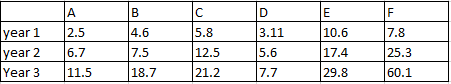### CAT 1996 Question Paper Question 5

Instructions

Direction for questions: Answer the questions based on the following information. The data given in the table shows the investment details in country ‘Fortune Land’ of companies A, B, C, D, E and F. Figures in the table are in US dollars in billions.Question 5

# By what percentage is the investments of companies D,E,F greater than the investments of companies A, B, C in year 2?

Solution

Investments of A, B and C = 6.7 + 7.5 + 12.5 = 26.7

Investments of A, B and C = 5.6 + 17.4 + 25.3 = 48.3

Difference = 21.6

% difference = $$\frac{21.6}{26.7}*100$$ = 80.8%